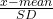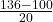## Assume that adults have IQ scores that are normally distributed with a mean of 100 and a standard deviation of 20. Find the probability that

Question

Assume that adults have IQ scores that are normally distributed with a mean of 100 and a standard deviation of 20. Find the probability that a randomly selected adult has an IQ less than 136.

in progress 0
2 months 2021-07-31T00:39:29+00:00 1 Answers 10 views 0

Randomly selected adult has an IQ less than 136 is 0.9641

Step-by-step explanation:

It is given that, it is normal distribution with mean 100 and SD as 20.

So, let’s use the formula of z-score

z=For this problem,

x= 136

Plug in this value into the formula

z-score==1.8

Now, use z-score table to find the probability

Find the corresponding value for the row 1.8 and the column 0.00, we do get 0.9641

So, Randomly selected adult has an IQ less than 136 is 0.9641